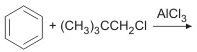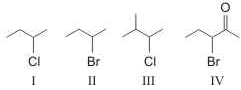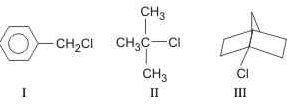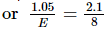Courses

# AIIMS Chemistry Mock Test - 8

## 60 Questions MCQ Test AIIMS Mock Tests & Previous Year Papers | AIIMS Chemistry Mock Test - 8

Description
This mock test of AIIMS Chemistry Mock Test - 8 for NEET helps you for every NEET entrance exam. This contains 60 Multiple Choice Questions for NEET AIIMS Chemistry Mock Test - 8 (mcq) to study with solutions a complete question bank. The solved questions answers in this AIIMS Chemistry Mock Test - 8 quiz give you a good mix of easy questions and tough questions. NEET students definitely take this AIIMS Chemistry Mock Test - 8 exercise for a better result in the exam. You can find other AIIMS Chemistry Mock Test - 8 extra questions, long questions & short questions for NEET on EduRev as well by searching above.
QUESTION: 1

Solution:
QUESTION: 2

Solution:
QUESTION: 3

### Which of the following is not a nucleophile?

Solution:
QUESTION: 4

The treatment of benzene with iso-butene in the presence of sulphuric acid gives

Solution:
QUESTION: 5

The structure of benzene is

Solution:
QUESTION: 6

Aromatic compounds undergo

Solution:
QUESTION: 7

Freon is used as a

Solution:
QUESTION: 8

The compound used as refrigerant is

Solution:
QUESTION: 9

The deecomposition of hydrogen peroxide 2H2O2 → 2H2O + O2 is a

Solution:
QUESTION: 10

Among the following, the paramagnetic compound is

Solution:
QUESTION: 11

An increase of temperature from 500 K to 510 K increases the number of bimolecular collisions by about__

Solution:
QUESTION: 12

Which one of the following statement is false?

Solution:
QUESTION: 13

In the following question, a Statement of Assertion (A) is given followed by a corresponding Reason (R) just below it. Read the Statements carefully and mark the correct answer-
Assertion(A): Ferrocene is an example of π-complex.
Reason(R): Iron and C5H5 ring double bond.

Solution:
QUESTION: 14

In the following question, a Statement-1 is given followed by a corresponding Statement-2 just below it. Read the statements carefully and mark the correct answer-

Statement-1: Glucose gives a reddish-brown precipitate with Fehling’s solution.
Statement-2: Reaction of glucose with Fehling’s solution gives CuO and gluconic acid.

Solution:
QUESTION: 15

In the following question, a Statement of Assertion (A) is given followed by a corresponding Reason (R) just below it. Read the Statements carefully and mark the correct answer-
Assertion(A): One mole of oxygen gas contains 2 x 6.023 x 1023 atoms of oxygen , whereas one mole of organ contains 6.023 x 1023.
Reason(R): Oxygen is a diatomic gas, O2 whereas argon is a monatomic gas.

Solution:
QUESTION: 16

In the following question, a Statement of Assertion (A) is given followed by a corresponding Reason (R) just below it. Read the Statements carefully and mark the correct answer-
Assertion(A): For the reaction
2N2O5 → 4NO2 + O2 ,
Rate = K[N2O5].
Reason(R): Rate of decomposition of N2O5 is determined by slow step.

Solution:
QUESTION: 17

In the following question, a Statement of Assertion (A) is given followed by a corresponding Reason (R) just below it. Read the Statements carefully and mark the correct answer-
Assertion(A): Oils contain higher proportion of glycerides of saturated fatty acids.
Reason(R): Oils contain a higher percentage of olein.

Solution:
QUESTION: 18

In the following question, a Statement of Assertion (A) is given followed by a corresponding Reason (R) just below it. Read the Statements carefully and mark the correct answer-
Assertion(A): LiCl is predominantly covalent in character.
Reason(R): Electronegativity difference between Li and Cl is too small.

Solution:
QUESTION: 19

In the following question, a Statement of Assertion (A) is given followed by a corresponding Reason (R) just below it. Read the Statements carefully and mark the correct answer-
Assertion(A): Para-hydrogen has lower internal energy and is favoured at high temperature.
Reason(R): The thermal conductivity of para-hydrogen is lesser than that of ortho-hydrogen.

Solution:
QUESTION: 20

In the following question, a Statement of Assertion (A) is given followed by a corresponding Reason (R) just below it. Read the Statements carefully and mark the correct answer-
Assertion(A): Benzene undergoes many electrophilic substitution reactions like acetylation.
Reason(R): The benzene ring rotates freely to form substituted compounds.

Solution:
QUESTION: 21

In the following question, a Statement of Assertion (A) is given followed by a corresponding Reason (R) just below it. Read the Statements carefully and mark the correct answer-
Assertion(A): During an adiabatic process, heat energy is not exchanged between system and surroundings.
Reason(R): The temperature of gas increases when it undergoes an adiabatic expansion.

Solution:
QUESTION: 22

In the following question, a Statement of Assertion (A) is given followed by a corresponding Reason (R) just below it. Read the Statements carefully and mark the correct answer-
Assertion(A): Phenol reacts with chloroform and aqueous alkali followed by acid hydrolysis to yield salicylaldehyde.
Reason(R): It involves the formation of carboncations.

Solution:
QUESTION: 23

In the following question, a Statement of Assertion (A) is given followed by a corresponding Reason (R) just below it. Read the Statements carefully and mark the correct answer-
Assertion(A): KMnO4 is purple in colour due to charge transfer.
Reason(R): MnO4- ion is tetrahedral in shape.

Solution:
QUESTION: 24

In the following question, a Statement of Assertion (A) is given followed by a corresponding Reason (R) just below it. Read the Statements carefully and mark the correct answer-
Assertion(A):In an acid-base titration involving strong base and a weak acid. methyl orange can be used as an indicator.
Reason(R):Methyl orange changes its colour in the pH range 3 to 5.

Solution:
QUESTION: 25

In the following question, a Statement of Assertion (A) is given followed by a corresponding Reason (R) just below it. Read the Statements carefully and mark the correct answer-
Assertion(A): Cu2+ is less stable than Cu+.
Reason(R): cu+ is diamagnetic .

Solution:
QUESTION: 26

In the following question, a Statement of Assertion (A) is given followed by a corresponding Reason (R) just below it. Read the Statements carefully and mark the correct answer-
Assertion(A): Lower members of aldehydes and ketones are miscible with water.
Reason(R): Solubility of carbonyl compounds increases with increase in molecular weight.

Solution:
QUESTION: 27

In the following question, a Statement-1 is given followed by a corresponding Statement-2 just below it. Read the statements carefully and mark the correct answer-
Statement-1:
Alkali metals dissolve in liquid ammonia to give blue solutions.
Statement-2:
Alkali metals in liquid ammonia give solvated species of the type [M(NH3)n]+ (M = alkali metals).

Solution:
QUESTION: 28

In the following question, a Statement of Assertion (A) is given followed by a corresponding Reason (R) just below it. Read the Statements carefully and mark the correct answer-
Assertion(A): Trans[Co(en)2Br2]Cl does not show optical activity.
Reason(R): Above coplex can exhibit ionisation isomerism.

Solution:
QUESTION: 29

In the following question, a Statement of Assertion (A) is given followed by a corresponding Reason (R) just below it. Read the Statements carefully and mark the correct answer-
Assertion(A):Nitrophenol is stronger acid than phenol.
Reason(R):Nitro group is electron withdrawing group and hence stabilises the anion formed.

Solution:
QUESTION: 30

In the following question, a Statement of Assertion (A) is given followed by a corresponding Reason (R) just below it. Read the Statements carefully and mark the correct answer-
Assertion(A): Alkylation of benzene by Friedal-Craft reaction gives polyalkylated compounds.
Reason(R): The ring gets activated for further substitution after the introduction of one alkyl group.

Solution:
QUESTION: 31

In the following question, a Statement of Assertion (A) is given followed by a corresponding Reason (R) just below it. Read the Statements carefully and mark the correct answer-
Assertion(A): Nitriding is a process of heating steel in an atmosphere of nitrogen.
Reason(R): Steel becomes brittle after nitriding.

Solution:
QUESTION: 32

In the following question, a Statement of Assertion (A) is given followed by a corresponding Reason (R) just below it. Read the Statements carefully and mark the correct answer-
Assertion(A): Mountaineers have to carry oxygen cylinders to avoid altitude sickness.
Reason (R): At altitudes as atmospheric pressure is low, air is less dense and less oxygen is available for breathing. this is explained by boyle's law.

Solution:
QUESTION: 33

A smuggler could not carry gold by chemically depositing iron on the gold surface since

Solution:
QUESTION: 34

When electricity is passed through aqueous solution of aluminium chloride, 13.5 g of Al are deposited. The number of faradays of electricity passed must be

Solution:
QUESTION: 35

A gas can be liquefied

Solution:
QUESTION: 36

Sodium metal cannot be stored under

Solution:
QUESTION: 37

Sodium nitrate decomposes above 800ºC to give

Solution:
QUESTION: 38

Which of the following imparts violet colouration to the Bunsen burner nonluminous flame?

Solution:
QUESTION: 39

Which of the following species has four lone pairs of electrons?

Solution:
QUESTION: 40

Plaster of Paris hardens by

Solution:
QUESTION: 41

Speed of decomposition of H₂O₂ is reduced by

Solution:
QUESTION: 42

The pH of blood does not appreciably change by a small addition of acid or a base because blood

Solution:
QUESTION: 43

A pH of 7 signifies

Solution:
QUESTION: 44

Which buffer solution out of the following will have pH > 7?

Solution:
QUESTION: 45

Which of the following is the strongest acid?

Solution:
QUESTION: 46

Acetone is easily oxidised with

Solution:
QUESTION: 47

The IUPAC name of acetone is

Solution:
QUESTION: 48

Intermediate electrophile in the following reaction is :Solution:
QUESTION: 49

Arrange these compounds in order of increasing SN2 reaction rate :Solution:
QUESTION: 50

E2 reaction is called:

Solution:
QUESTION: 51

To obtain pure silicon, SiH₄ is heated at

Solution:
QUESTION: 52

Ultra-silicon is prepared by the process of

Solution:
QUESTION: 53

The edge length of face centred unit cubic cell is 508 pm. If the radius of the cation is 110 pm, the radius of the anion is

Solution:
QUESTION: 54

There is formation of precipitate almost instantly when alcoholic AgNO3 reacts withSolution:
QUESTION: 55

At 25oC, the highest osmotic pressure is exhibited by 0.1 M solution of

Solution:
QUESTION: 56

Volume of 0.1 M K₂Cr₂O₇ required to oxidise 35 ml of 0.5 M FeSO₄ solution is

Solution:
QUESTION: 57

At room temperature, the mole fraction of a solution is 0.25 and the vapour pressure of the solvent is 0.80 atm. Then the lowering of vapour pressure is

Solution:
QUESTION: 58

A metal oxide is reduced by heating it in a stream of hydrogen. It is found that after complete reduction, 3.15 g of the oxide has yielded 1.05 g of the metal. We may deduce that

Solution:

Equivalent of metal = Equivalent of oxygenor E = 4.

QUESTION: 59

On addition of NH₄OH to a copper sulphate solution?

Solution:
QUESTION: 60

Which diagram describes the energy changes for rotation about the 2 - 3 bond of 2, 3 - dimethyl butane?

Solution: